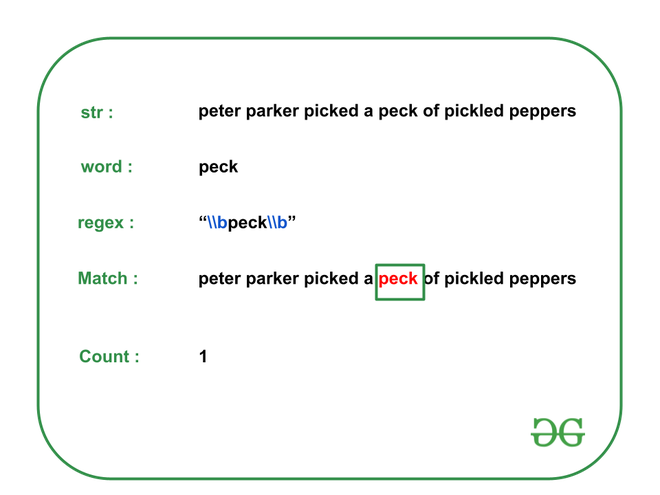# Count occurrences of a word in string | Set 2 (Using Regular Expressions)

• Last Updated : 31 Mar, 2022

Given a string str and a word w, the task is to print the number of the occurrence of the given word in the string str using Regular Expression.

Examples:

Input: str = “peter parker picked a peck of pickled peppers”, w = “peck”
Output: 1
Explanation: There is only one occurrence of the word “peck” in the given string. Therefore, the output is 1.Input: str = “How much wood would a woodchuck chuck if a woodchuck could chuck wood ?”, w = “wood”
Output: 2
Explanation: There are only two occurrences of the word “wood” in the given string.
Therefore, the output is 2.

Input: str = “She sells seashells by the seashore”, w = “sea”
Output: 0
Explanation: There is no occurrence of the word “sea” in the given string. Therefore, the output is 0.

Approach: The required regular expression to find the required count of string w in the given string is “\\bw\\b”, where \b is a word boundary. Follow the steps to solve the problem

regex = “\\bw\\b”

Below is the implementation of the above approach :

## C++

 `// C++ program for the above approach``#include ``#include ``using` `namespace` `std;` `// Function to count total occurrences``// of word "w" in string "str"``void` `countOccurrences(string str, string w)``{``    ``// Get the regex to be checked``    ``string regexPattern = ``"\\b"` `+ w + ``"\\b"``;``    ``const` `regex pattern(regexPattern);` `    ``// Variable to count total``    ``// occurrences of the given word``    ``int` `count = 0;` `    ``auto` `it``        ``= sregex_iterator(str.begin(), str.end(), pattern);` `    ``for` `(it; it != sregex_iterator(); it++) {` `        ``// Increment count``        ``count++;``    ``}` `    ``// Print the occurrences of the word``    ``cout << count << endl;` `    ``return``;``}` `// Driver Code``int` `main()``{``    ``// Input``    ``string str``        ``= ``"peter parker picked a peck of pickled peppers"``;``    ``string w = ``"peck"``;` `    ``countOccurrences(str, w);` `    ``return` `0;``}`

## Java

 `// Java program for the above approach``import` `java.util.*;``import` `java.util.regex.*;` `class` `GFG {` `  ``// Function to count total occurrences``  ``// of word "w" in String "str"``  ``static` `void` `countOccurrences(String str, String w)``  ``{``    ` `    ``// Get the regex to be checked``    ``String regexPattern = ``"\\b"` `+ w + ``"\\b"``;` `    ``Pattern pattern = Pattern.compile(regexPattern);``    ` `    ``// Variable to count total``    ``// occurrences of the given word``    ``int` `count = ``0``;` `    ``while` `(str.contains(w)) {``      ``str = str.replace(w, ``""``);``      ``// Increment count``      ``count++;``    ``}` `    ``// Print the occurrences of the word``    ``System.out.print(count + ``"\n"``);` `    ``return``;``  ``}` `  ``// Driver Code``  ``public` `static` `void` `main(String[] args)``  ``{``    ` `    ``// Input``    ``String str = ``"peter parker picked a peck of pickled peppers"``;``    ``String w = ``"peck"``;` `    ``countOccurrences(str, w);``  ``}``}` `// This code is contributed by gauravrajput1`

## C#

 `// C# program for the above approach``using` `System;``using` `System.Text.RegularExpressions;``public` `class` `GFG {` `  ``// Function to count total occurrences``  ``// of word "w" in String "str"``  ``static` `void` `countOccurrences(String str, String w)``  ``{` `    ``// Get the regex to be checked``    ``String regexPattern = ``"\\b"` `+ w + ``"\\b"``;` `    ``Regex rx = ``new` `Regex(regexPattern,RegexOptions.Compiled | RegexOptions.IgnoreCase);``    ``// Variable to count total``    ``// occurrences of the given word``    ``int` `count = 0;` `    ``while` `(str.Contains(w)) {``      ``str = str.Replace(w, ``""``);``      ``// Increment count``      ``count++;``    ``}` `    ``// Print the occurrences of the word``    ``Console.Write(count + ``"\n"``);` `    ``return``;``  ``}` `  ``// Driver Code``  ``public` `static` `void` `Main(String[] args)``  ``{` `    ``// Input``    ``String str = ``"peter parker picked a peck of pickled peppers"``;``    ``String w = ``"peck"``;` `    ``countOccurrences(str, w);``  ``}``}` `// This code is contributed by gauravrajput1`

Output

`1`

Time Complexity : O(N)
Auxiliary Space : O(N)

My Personal Notes arrow_drop_up